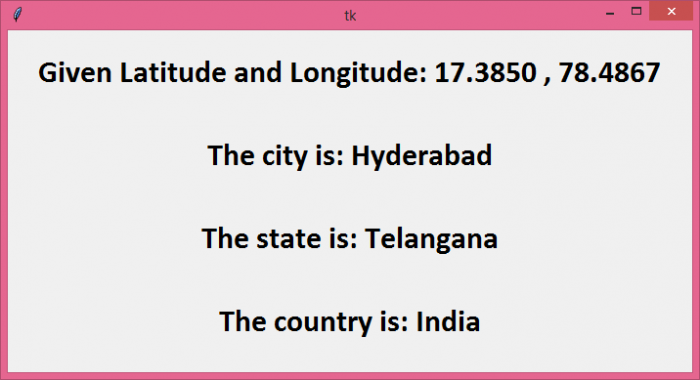# How to get the longitude and latitude of a city using Python?

To get the longitude and latitude of a city, we will use the geopy module. geopy uses third-party geocoders and other data sources to locate the coordinates of addresses, cities, countries, etc.

First of all, make sure the geopy module is installed −

pip install geopy

In the following example, we will use the Nominatim geocoder to find the longitude and latitude of the city "Hyderabad".

## Steps −

• Import Nominatim geocoder from geopy module.

• Initialize the Nominatim API and use the geocode method to get the location of the input string.

• Finally, get the latitude and longitude of the location by location.latitude and location.longitude.

## Example 1

# Import the required library
from geopy.geocoders import Nominatim

# Initialize Nominatim API
geolocator = Nominatim(user_agent="MyApp")

print("The latitude of the location is: ", location.latitude)
print("The longitude of the location is: ", location.longitude)

## Output

It will print the following output on the console −

The latitude of the location is: 17.360589
The longitude of the location is: 78.4740613

In this example, let's do the opposite of Example 1. We will start by providing a set of coordinates and find the city, state, and country those coordinates represent. Instead of printing the output on the console, we will create a tkinter window with four labels to display the output.

## Steps −

• Initialize the Nominatium API.

• Use the geolocator.reverse() function and supply the coordinates (latitude and longitude) to get the location data.

• Get the address of the location using location.raw['address'] and traverse the data to find the city, state, and country using address.get().

• Create labels inside a tkinter window to display the data.

## Example 2

from tkinter import *
from geopy.geocoders import Nominatim

# Create an instance of tkinter frame
win = Tk()

# Define geometry of the window
win.geometry("700x350")

# Initialize Nominatim API
geolocator = Nominatim(user_agent="MyApp")

# Latitude & Longitude input
coordinates = "17.3850 , 78.4867"

location = geolocator.reverse(coordinates)

# Traverse the data

# Create a Label widget
label1=Label(text="Given Latitude and Longitude: " + coordinates, font=("Calibri", 24, "bold"))

label2=Label(text="The city is: " + city, font=("Calibri", 24, "bold"))

label3=Label(text="The state is: " + state, font=("Calibri", 24, "bold"))

label4=Label(text="The country is: " + country, font=("Calibri", 24, "bold"))
win.mainloop()